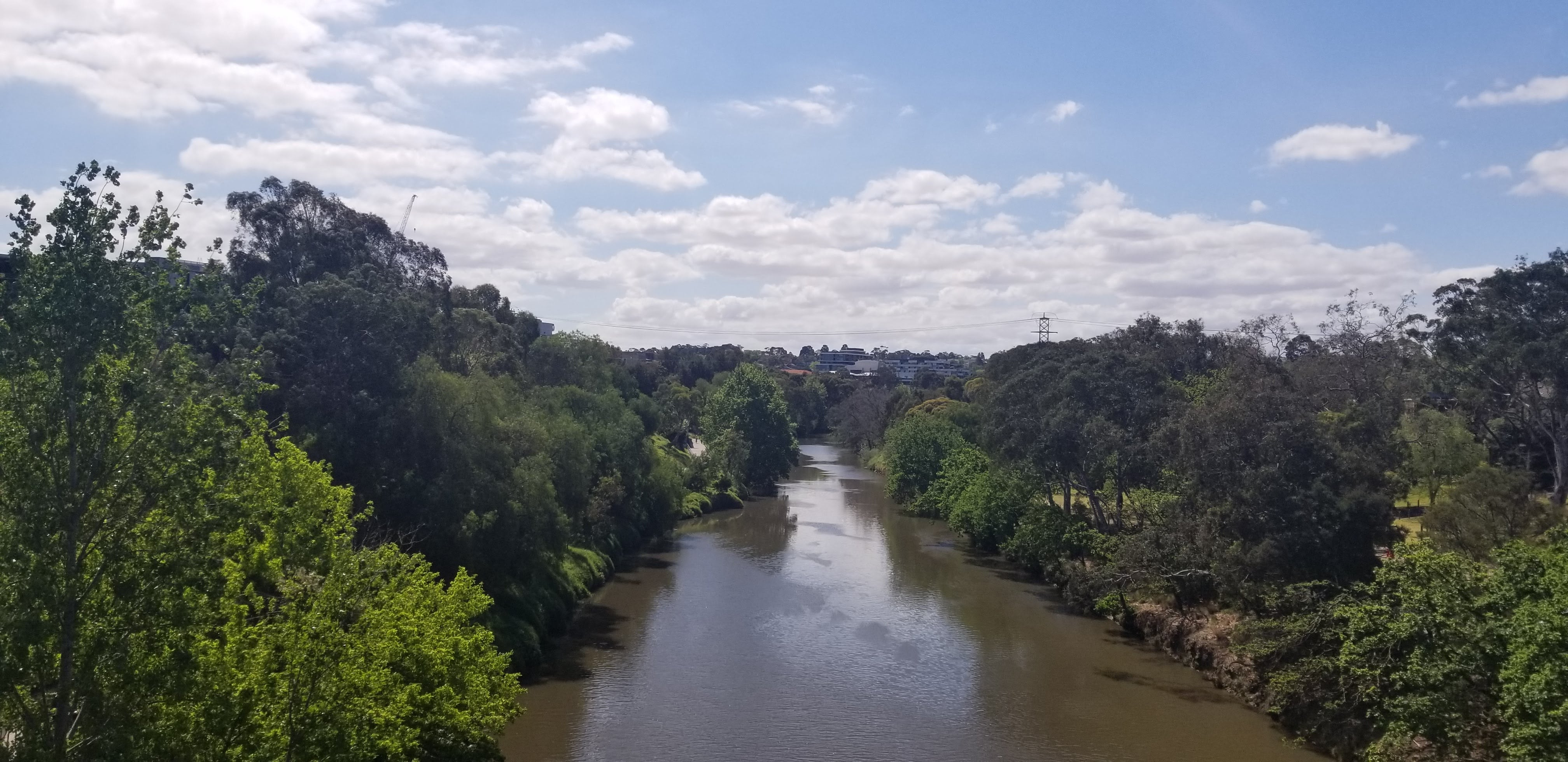180 degree southThe best journey answers the question that in the beginning you didn’t even think to ask

BERT based sentence scenario detector

# 模型构建
model = Sequential([
Conv1D(filters=5, kernel_size=5, strides=1, padding='valid', input_shape=(768, 1), name="Convolution_Layer_1"),
AveragePooling1D(pool_size=5, strides=1, padding="valid", name="Pooling_Layer_1"),

Conv1D(filters=5, kernel_size=5, strides=1, padding='valid', name="Convolution_Layer_2"),
AveragePooling1D(pool_size=5, strides=1, padding="valid", name="Pooling_Layer_2"),

Flatten(name="Flatten_Layer"),

Dense(256, input_dim=3760, name="Dense_Layer_1"),
Activation('relu'),
Dropout(0.1),

Dense(32, input_dim=256, name="Dense_Layer_2"),
Activation('relu'),
Dropout(0.1),

Dense(11, input_dim=32, name="Dense_Layer_3"),
Activation('softmax'),
])

通用场景识别器

# 模型构建
model = Sequential([
Dense(32, input_dim=200),
Activation('relu'),
Dropout(0.1),

Dense(16, input_dim=32),
Activation('relu'),

Dense(9, input_dim=32),
Activation('softmax'),
])

test loss:  0.09276254528926478
test accuracy: 0.9666666666666667